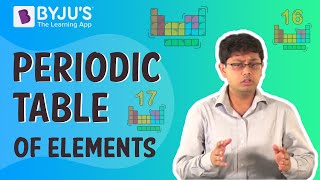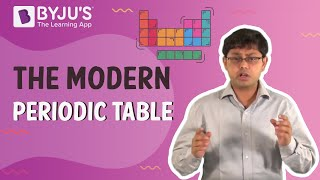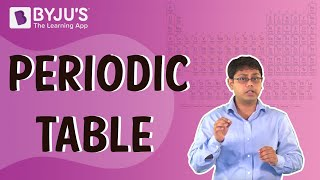Jet Set Go! All about Aeroplanes Jet Set Go! All about Aeroplanes

# Modern Periodic Table Questions

Henry Moseley proposed the modern periodic table in 1913. He states that the properties of elements are a periodic function of their atomic numbers. He placed the elements in distinct rows and columns. The rows were called periods, and columns were called groups. There are seven rows and eighteen columns in the modern periodic table.

 Definition: The modem periodic law of classification of elements states that the properties of elements are a periodic function of their atomic numbers.

## Modern Periodic Table Chemistry Questions with Solutions

Q1. Which of the following group of the modern periodic table contains halogen?

(a) Group 1

(b) Group 2

(c ) Group 17

(d) None of the above

Answer: (c ) Group 17 of the modern periodic table contains halogens.

Q2. Which of the following is the most electropositive halogen?

(a) Fluorine

(b) Chlorine

(c ) Bromine

(d) None of the above

Answer: (c ) Bromine is the most electropositive halogen.

Explanation: Fluorine, chlorine, and bromine are the same group members. Fluorine is placed at the top of the modern periodic table, while bromine is present at the lower end of the modern periodic table. As we move down in the modern periodic table, the size of the atom increases, decreasing the effective nuclear charge, Thereby increasing the tendency to donate electrons. Thus, electropositivity rises as we move down in the modern periodic table.

Hence, bromine is the most electropositive halogen.

Q3. Periods IInd elements are typically known as

(a) Alkali Metal

(b) Alkaline earth metals

(c ) Bridged elements

(d) None of the above

Answer: (c ) Periods IInd elements are typically known as bridged elements.

Q4. How does the metallic character of elements change when we go up in the modern periodic table?

(a) Increases

(b) Decreases

(c ) Remains the same

(d) First decreases then increases

Answer: (b) Metallic character of elements decreases as we go up in the modern periodic table.

Explanation: As we go up in the modern periodic table, the size of the atom decreases, increasing the effective nuclear charge. Thus, the tendency to lose electrons decreases as we move up.

Hence, the metallic character of an element decreases as we move up in the modern periodic table.

Q5. Which of the following non-metals exist in a liquid state at room temperature?

(a) Mercury

(b) Bromine

(c ) Iodine

(d) None of the above

Answer: (b) Bromine exists in a liquid state at room temperature.

Q6. Name two elements whose properties were predicted based on their positions in Mendeleev’s periodic table. Write the formulae of their chlorides.

Answer: Eka-aluminium, i.e. Gallium and Eka-silicon, i.e. Germanium, are the two elements whose properties were predicted based on their positions in Mendeleev’s periodic table.

The formula of chloride of gallium is GaCl3, and germanium is GeCl4.

Q7. Arrange the following elements in the increasing order of their metallic character.

Mg, Ca, K, Ge, Ga

Answer: We can arrange Mg, Ca, K, Ge, Ga as Ge < Ga Mg < Ca < K.

Q8. An element X is in Group II of the modern periodic table:

(а) What will its chloride formula be?

(b) What will its oxide formula be?

Answer: The element of group II of the modern periodic table has valency 2.

(a) The chloride formula of group II element X of the modern periodic table will be XCl2.

(b) The oxide formula of group II element X of the modern periodic table will be XO.

Q9. An element ‘Y’ with the atomic number 3 combines with an element ‘A’ with the atomic number 17. What will be the formula of its compound?

Answer: The element with atomic number 3 is lithium, while an element with atomic number 17 is chlorine. The formula of their compound will be LiCl.

Q10. Why hydrogen occupies a unique position in the Modern Periodic Table.

Answer: Hydrogen occupies a unique position in the Modern Periodic Table because of its unique electronic configuration 1. It resembles alkali metal, as all the alkali metal has one valence electron, and by losing one electron, it acquires a stable nobel configuration. Moreover, it also resembles halogens. Like halogens, it gains one electron to acquire a stable nobel configuration.

Thus, hydrogen occupies a unique position in the Modern Periodic Table.

Q11. What is the modem periodic law of classification of elements?

Answer: The modem periodic law of classification of elements states that the properties of elements are a periodic function of their atomic numbers.

Q12. Lithium, sodium and potassium form a Dobereiner’s triad. The atomic masses of lithium and potassium are 7 and 39, respectively. Expect the atomic mass of sodium.

Answer: As Lithium, sodium and potassium form a Dobereiner’s triad. Then the mean of atomic masses of lithium and potassium must be equal to the atomic mass of sodium.

The atomic mass of Sodium = (Atomic mass of lithium + Atomic mass of potassium) / 2

The atomic mass of Sodium = (7 + 39) / 2

The atomic mass of Sodium = 46 / 2

The atomic mass of Sodium = 23.

Q13. In the modern periodic table, the element Calcium with atomic number 20 is surrounded by elements with atomic numbers 12, 19, 21 and 38. Which of these elements will have physical and chemical properties corresponding to Calcium? Give reason.

Answer: To predict the elements with physical and chemical properties corresponding to Calcium, we will write the electronic configuration of elements with atomic numbers 12, 19, 20, 21 and 38.

 Atomic Number Electronic Configuration 12 2, 8, 2 19 2, 8, 8, 1 20 2, 8, 8, 2 21 2, 8, 9, 2 38 2, 8, 18, 8, 2

The electronic configuration of the element with atomic number 12 and 38 resembles with that of calcium as they all have same valence electrons. Thus, it will have physical and chemical properties corresponding to Calcium.

Q14. An element ‘M’ has the atomic number 11.

(a) Write the electronic configuration of element M.

(b) State the group to which ‘M’ belongs.

(c) Is ‘M’ a metal or a non-metal?

(d) Write the chloride formula of element M.

Answer: The element with atomic number 11 is sodium.

(a) The electronic configuration of element M is 2, 8, 1.

(b) ‘M’ belongs to group Ist of the modern periodic table.

(c) ‘M’ is a metal.

(d) The chloride formula of element M is MCl.

Q15. Match the following.

 Column 1 Column 2 Be 74 C 88 O 111 B 77 N 66

 Column 1 Column 2 Be 111 C 66 O 77 B 88 N 74

## Practise Questions on Modern Periodic Table

Q1. Distinguish between electron gain enthalpy and electronegativity.

Q2. How does electronic configuration affect the position of an element in the modern periodic table?

Q3. An element ‘X’ belongs to the 3rd period and group 17 of the modern periodic table. State its

(a) Electronic configuration

(b) Valency.

Q4. The formula of magnesium oxide is MgO. State the barium nitrate and barium sulphate formula if barium belongs to the same group as magnesium.

Q5. What are the various factors that affect the electron gain enthalpy of a molecule?

Click the PDF to check the answers for Practice Questions.

## Recommended Videos

#### Periodic Table of Elements#### Periodic Table – The Modern Periodic Table | Learn with BYJU’S#### Periodic Table – Introduction#### Periodic Table 04 – Be More Curious